## RD Sharma Class 9 Solutions Chapter 19 Surface Areas and Volume of a Circular Cylinder Ex 19.1

These Solutions are part of RD Sharma Class 9 Solutions. Here we have given RD Sharma Class 9 Solutions Chapter 19 Surface Areas and Volume of a Circular Cylinder Ex 19.1

Other Exercises

Question 1.
Curved surface area of a right circular cylinder is 4.4 m2. If the radius of the base of the cylinder is 0.7 m, find its height. [NCERT]
Solution:
Curved surface area of a cylinder = 4.4 m2
Radius (r) = 0.7 m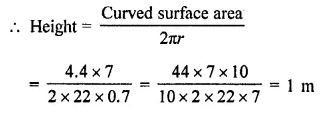Question 2.
In a hot water heating system, there is a cylindrical pipe of length 28 m and diameter 5 cm. Find the total radiating surface in the system. [NCERT]
Solution:
Diameter of the pipe = 5 cm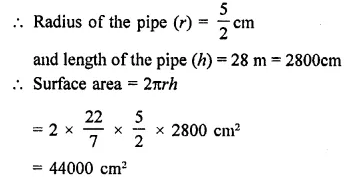Question 3.
A cylindrical pillar is 50 cm in diameter and 3.5 m in height. Find the cost of painting the curved surface of the pillar at the rate of 12.50 per m2. [NCERT]
Solution:
Diameter of cylindrical pillar = 50 cm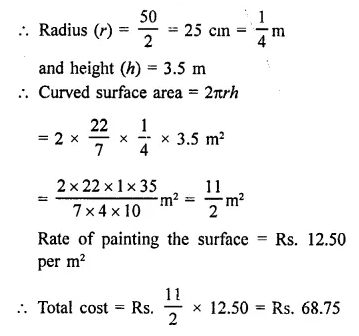Question 4.
It is required to make a closed cylindrical tank of height 1 m and base diameter 140 cm from a metal sheet. How many square metres of the sheet are required for the same? [NCERT]
Solution:
Height of cylinder (h) = 1 m = 100 cm
Diameter of box = 140 cm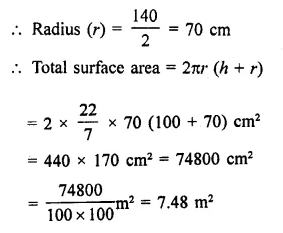Question 5.
The total surface area of a hollow cylinder which is open from both sides is 4620 sq. cm, area of base ring is 115.5 sq. cm and height 7 cm. Find the thickness of the cylinder.
Solution:
Total surface area of a hollow cylinder open from both sides = 4620 cm2
Area of base of ring = 115.5 cm2
Height (h) = 7 cm
Let outer radius (R) = R
and inner radius = rQuestion 6.
Find the ratio between the total surface area of a cylinder to its curved surface area, given that its height and radius are 7.5 cm and 3.5 cm.
Solution:
Radius of the cylinder (r) = 3.5 cm
and height (h) = 7.5 cm
Total surface area = 2πr (h + r)
and curved surface area = 2πrhQuestion 7.
A cylindrical vessel, without lid, has to be tin-coated on its both sides. If the radius of the base is 70 cm and its height is 1.4 m, calculate the cost of tin-coating at the rate of ₹3.50 per 1000 cm2.
Solution:
Radius of the base of a cylindrical vessel (r) = 70 cm
and height (h) = 1.4 m = 140 cm
Total surface area (excluding upper lid) on both sides = 2πrh x 2 + πr2 x 2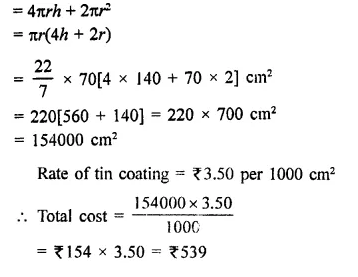Question 8.
The inner diameter of a circular well is 3.5 m. It is 10 m deep. Find:
(i) inner curved surface area.
(ii) the cost of plastering this curved surface at the rate of ₹40 per m2. [NCERT]
Solution:
Inner diameter of a well = 3.5 m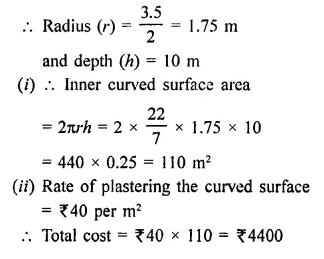Question 9.
The students of a Vidyalaya were asked to participate s a competition for making and decorating pen holders in the shape of a cylinder with a base, using cardboard. Each pen holder was to be of radius 3 cm and height 10.5 cm. The Vidyalaya was to supply the competitors with cardboard. If there were 35 competitors, how much cardboard was required to be bought for the competition? [NCERT]
Solution:
Radius of cylinderical pen holder (r) = 3 cm
Height (h) = 10.5 cm
∴ Surface area of the pen holder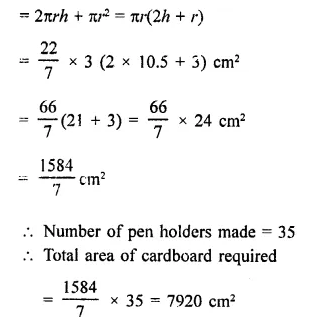Question 10.
The diameter of roller 1.5 m long is 84 cm. If it takes 100 revolutions to level a play¬ground, find the cost of levelling this ground at the rate of 50 paise per square metre.
Solution:
Diameter of a roller = 1.5 m
∴ Radius = $$\frac { 1.5 }{ 2 }$$ = 0.75 m = 75 cm
and length (h) = 84 cm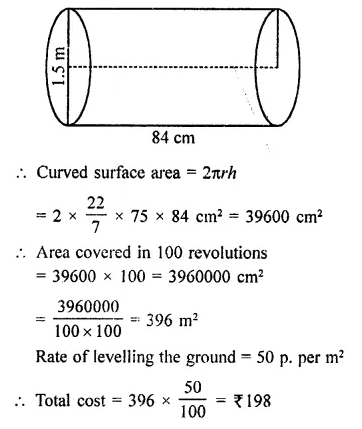Question 11.
Twenty cylindrical pillars of the Parliament House are to be cleaned. If the diameter of each pillar is 0.50 m and height is 4 m. What will be the cost of cleaning them at the rate of ₹2.50 per square metre? [NCERT]
Solution:
Number of pillars = 20
Diameter of one pillar = 0.50 m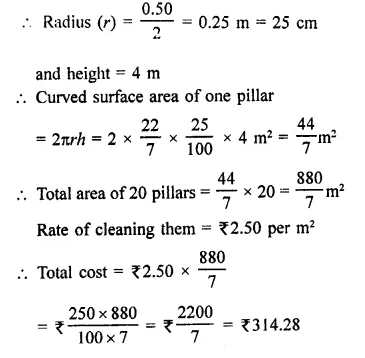Question 12.
A solid cylinder has total surface area of 462 cm2. Its curved surface area is one- third of its total surface area. Find the radius and height of the cylinder.
Solution:
Total surface of solid cylinder = 462 cm2
Curved surface area = $$\frac { 1 }{ 3 }$$ of total surface areaQuestion 13.
The total surface area of a hollow metal cylinder, open at both ends of external radius 8 cm and height 10 cm is 338 π cm2. Taking r to be inner radius, obtain an equation in r and use it to obtain the thickness of the metal in the cylinder.
Solution:
Total surface area of a hollow metal cylinder = 338π cm2
Let R be the outer radius, r be inner radius and h be the height of the cylinder of the cylinder
∴ 2πRh + 2πrh + 2πR2 – 2πr2 = 338π
R = 8 cm, h = 10 cm
⇒ 2πh (R + r) + 2π(R2 – r2) = 338π
⇒ Dividing by 2π , we get
⇒ h(R + r) + (R2 – r2) = 169
⇒ 10(8 + r) + (8 + r) (8 – r) = 169
⇒ 80 + 10r + 64 – r2 = 169
⇒ 10r – r2 + 144 – 169 = 0
⇒ r2 – 10r + 25 = 0
⇒ (r-5)2 = 0
⇒ r = 5
∴ Thickness of the metal = R – r = 8 – 5 = 3 cm

Question 14.
Find the lateral curved surface area of a cylinderical petrol storage tank that is 4.2m in diameter and 4.5 m high. How much steel was actually used, if $$\frac { 1 }{ 12 }$$ of steel actually used was wasted in making the closed tank? [NCERT]
Solution:
Diameter of a cylinderical tank = 4.2 m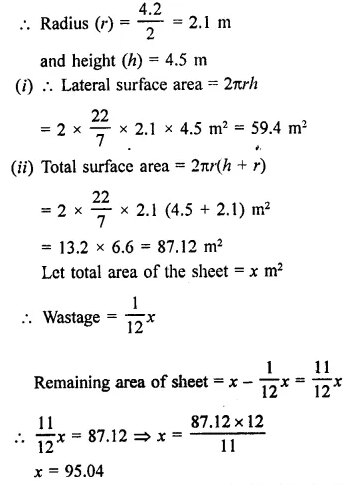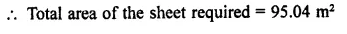Hope given RD Sharma Class 9 Solutions Chapter 19 Surface Areas and Volume of a Circular Cylinder Ex 19.1 are helpful to complete your math homework.

If you have any doubts, please comment below. Learn Insta try to provide online math tutoring for you.